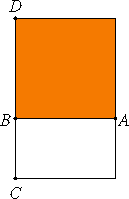# Proposition 21

The rectangle contained by rational straight lines commensurable in square only is irrational, and the side of the square equal to it is irrational. Let the latter be called medial.

Let the rectangle AC be contained by the rational straight lines AB and BC commensurable in square only.I say that AC is irrational, and the side of the square equal to it is irrational, and let the latter be called medial.

X.Def.I.4

Describe the square AD on AB. Then AD is rational.

And, since AB is incommensurable in length with BC, for by hypothesis they are commensurable in square only, while AB equals BD, therefore DB is also incommensurable in length with BC.

And DB is to BC as AD is to AC, therefore DA is incommensurable with AC.

X.Def.I.4

But DA is rational, therefore AC is irrational, so that the side of the square AC is also irrational.

Let the latter be called medial.

Q.E.D.

## Guide

The two lines AB and BC are rational lines. That means, according to Definition 3, that each is commensurable in square to some unmentioned, standard straight line. It’s convenient to assign that standard line a length of 1 and the standard square on it a square of area 1. Euclid did not, but doing so allows us to assign lengths to those lines. So if a is the length of AB, that means a2 is a rational number, that is, a ratio of two whole numbers. Likewise, if b is the length of BC, then b2 is a rational number.

Now AB and BC are also commensurable in square only. First of all, they’re commensurable in square, so the squares on them are in a numeric ratio, and that means a2/b2 is a rational number, which we already know since both a2 and b2 are rational numbers themselves. But they’re also commensurable in square only. That means that a/b is an irrational number. In summary, a2 and b2 are rational, but a/b is irrational.

In the proof, it’s noted that the rectangle AC (which is a a by b rectangle), is irrational, that is, ab is an irrational number. The side of a square equal equal to that rectangle is also irrational, and it’s called medial. The length of that side is √(ab).

Another way of saying that is that the length √(ab) of a medial line is the geometric mean of two numbers a and b whose squares are rational and whose ratio is irrational.

#### Example and interpretation

Let AB be the standard line and BC be the diagonal of the standard square, then √(ab) = √(√2), the 4th root of 2.

In fact, all medial lines have lengths 4th roots of rational numbers whose square roots are irrational. If a2 and b2 are rational numbers but a/b is an irrational number, then √(ab) is a number whose fourth power, a2b2, is a rational number but whose square, ab, is an irrational number.

Thus, we could call a number whose square is an irrational number but whose fourth power is a rational number a medial number.

#### Medial squares and rectangles

Only medial lines were defined in this proposition, but the adjective medial is applied to squares and rectangles starting with Proposition X.24. From the way it is used, we can tell that a medial square is one whose side is a medial line, and a medial rectangle is one equal to a square whose side is a medial line.

#### Use of this proposition.

This proposition is used frequently in Book X starting with the next proposition.